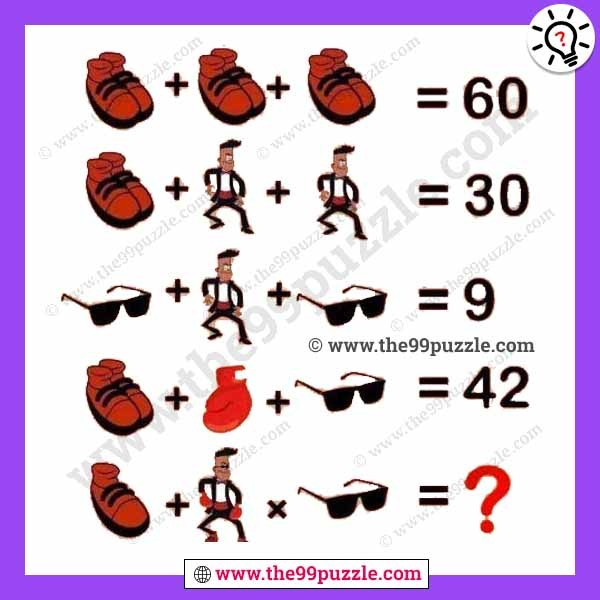# Shoes Man Specs Boxing Glove Equation Puzzle – Puzz136

Shoes Man Specs Boxing Glove Equation Puzzle. This viral picture puzzle stumped the internet. This brainteaser picture only solves the genius people. Observe this picture carefully and calculate the value of the last equation Shoes Man Specs Boxing Glove puzzle.###### Explanation:

(10+10)+(10+10)+(10+10)=60

(10+10)+5+5=30

2+5+2=9

(10+10)+20+2=42

10+(5+10+10+20+20+2)×2=144